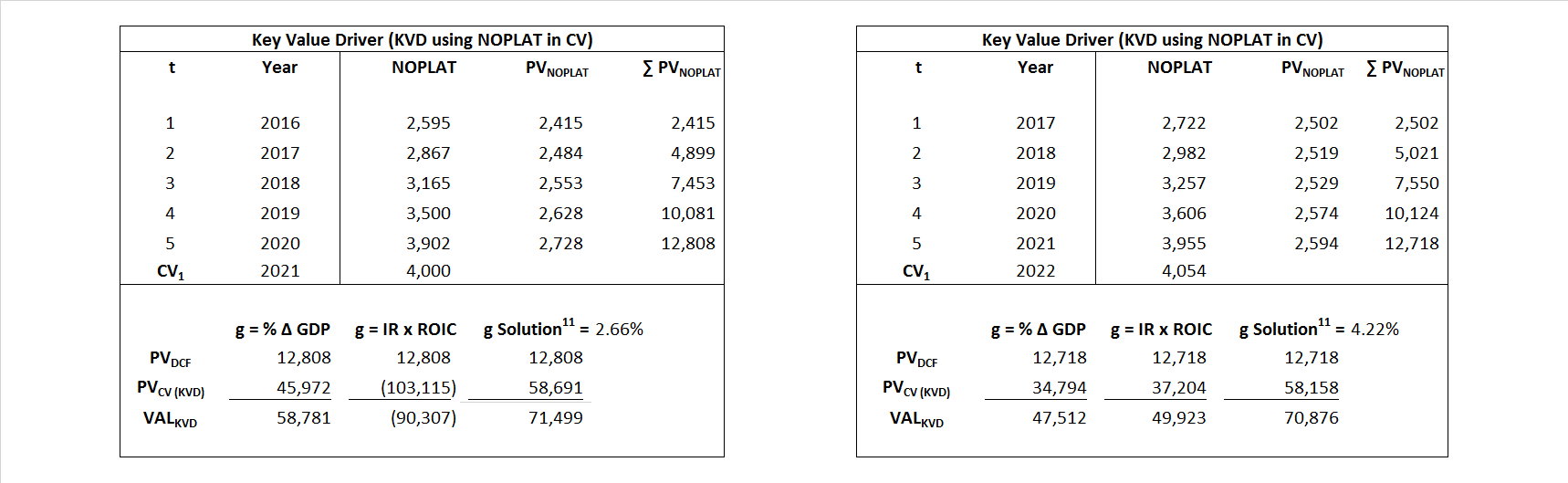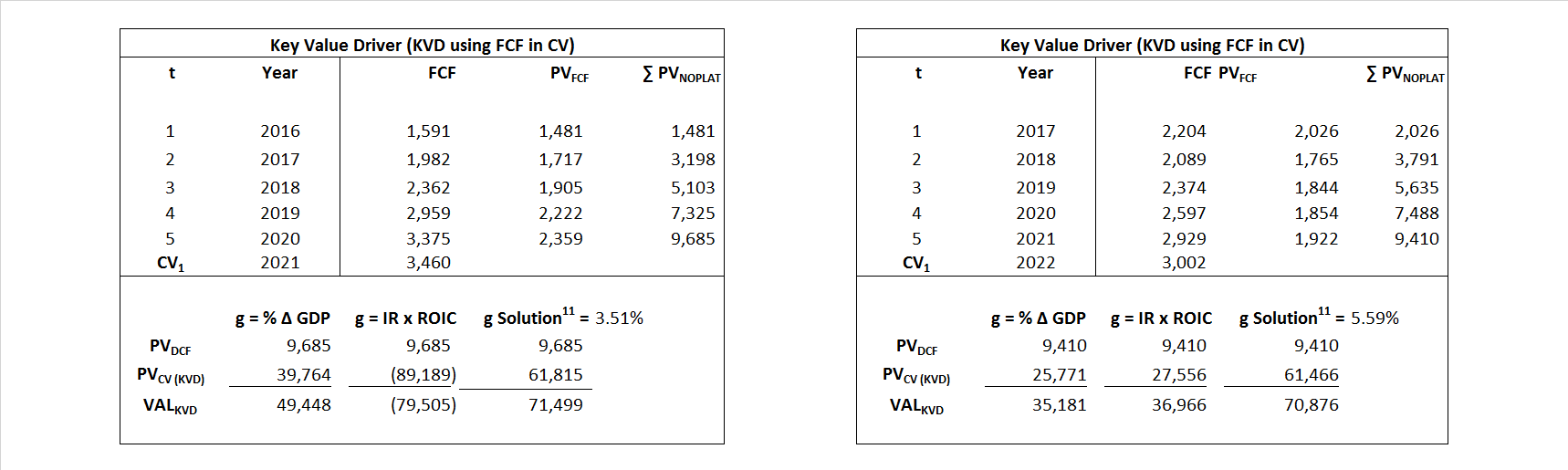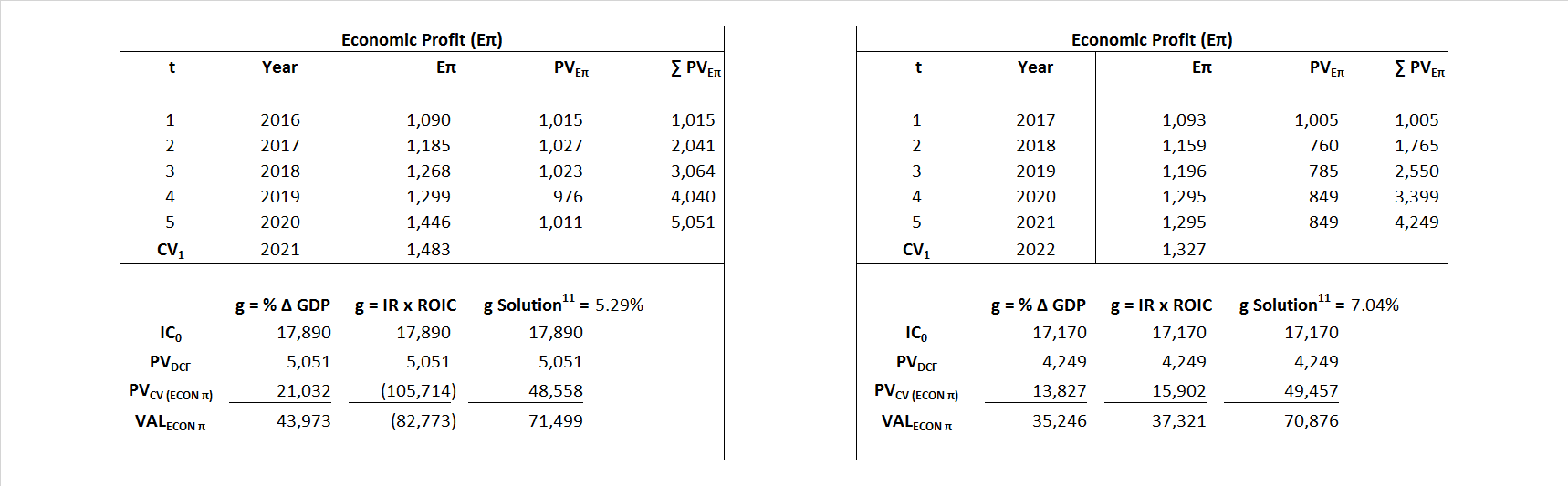# Costco

## Analyst Listing

The following analysts provide coverage for the subject firm as of May 2016:

 Broker Analyst Analyst Email Stephens Inc Ben Wyatt ben.wyatt@stephens.com Susquehanna Financial Group Biju Perincheril biju.perincheril@sig.com Johnson Rice & Company Charles Meade cmeade@jrco.com Cowen & Company Charles Robertson charles.robertson@cowen.com Piper Jaffray David Kistler david.w.kistler@simmonspjc.com Wells Fargo Securities David Tameron david.tameron@wellsfargo.com GMP Securities Derrick Whitfield dwhitfield@gmpsecurities.com Credit Suisse Edward Westlake edward.westlake@credit-suisse.com IBERIA Capital Partners Eric Fox eric.fox@iberiabank.com Evercore ISI Greg Melich greg.melich@evercoreisi.com Alembic Global Advisors James Sullivan james.sullivan@alembicglobal.com KLR Group John Gerdes jjg@klrgroup.com Jefferies Jonathan D. Wolff jwolff@jefferies.com Telsey Advisory Group Joseph Feldman jfeldman@telseygroup.com Raymond James Kevin Smith kevin.smith@raymondjames.com Nomura Research Lloyd Byrne lloyd.byrne@nomura.com Seaport Global Securities Michael Kelly mkelly@seaportglobal.com Mitsubishi UFJ Securities (USA) Michael McAllister mmcallister@us.sc.mufg.jp Wolfe Research Paul Sankey psankey@wolferesearch.com Scotia Howard Weil Peter Kissel peter.kissel@scotiabank.com BMO Capital Markets Phillip Jungwirth phillip.jungwirth@bmo.com Capital One Securities Phillips Johnston phillips.johnston@capitalone.com Deutsche Bank Research Ryan Todd ryan.todd@db.com RBC Capital Markets Scott Hanold scott.hanold@rbccm.com Evercore ISI Stephen Richardson stephen.richardson@evercoreisi.com Guggenheim Securities Subash Chandra subash.chandra@guggenheimpartners.com Wunderlich Securities Vedran Vuk vedranvuk@wundernet.com Roth Capital Partners Chris Lewis clewis@roth.com The Benchmark Company, LLC Jan Wald jwald@benchmarkcompany.com Canaccord Genuity Jason Mills jmills@canaccordgenuity.com Brean Capital, LLC Jason Wittes jwittes@breancapital.com Barrington Research Michael Petusky mpetusky@brai.com Stifel Nicolaus Rick Wise wiser@stifel.com

## Primary Input Data## Derived Input Data

### Equational Form

Net Operating Profit Less Adjusted Taxes NOPLAT   2,356   2,438$NOPLAT\, =\, EBIT\, x\, (1 \,-\, Avg \,\,Tax\,\, Rate\,\, on\,\, EBIT)$
Free Cash Flow FCF  1,375  2,610$FCF\,=NOPLAT\,+\,Non-Cash\,Expenses-\Delta NWC\,-\,NCS$
Tax Shield TS  41  44$TS\,=\,Interest\,\,Paid\,\,x\,\, Avg \,\,Tax\,\,Rate\,\, on\,\, Pre-Tax\,\, Income$
Invested Capital IC  17,890  17,170$IC\,=\,Fixed\,\,Operating\,\,Assets\,\,+\,\,Net\,\, Working\,\, Capital$
Return on Invested Capital ROIC 13.17% 14.20%$ROIC\,=\,\frac { NOPLAT }{ IC }$
Net Investment NetInv  1,504  570$NetInv\,=\,{ {IC}_{1}}-{{IC}_{0}}+Depreciation$
Investment Rate IR 63.85% 23.40%$IR\,=\,\frac {NetInv}{NOPLAT}$
Weighted Average Cost of Capital WACCMarket 7.43% 8.80%$WACC\,=\,\frac { E }{ V } { R }_{ E }\,+\,\frac { P }{ V } { R }_{ P }\,+\,\frac { D }{ V } { R }_{ D }\left( 1- Avg\,\, Tax\,\,Rate\,\,on\,\,Pre-Tax\,\,Income \right)$
WACCBook  7.39% 8.07%
Enterprise value EVMarket  71,499  70,876$EV\,=\,Market\,\,Cap\,\,Equity\,+\,\,Long\,\,Term\,\,Debt\,-\,Cash$
EVBook  70,459  70,749
EV/EBIT Multiple$\frac{EV_{Market}}{EBIT}$  19.73  18.90$EV/EBIT\,=\,\frac { EV}{ EBIT}$
Long-Run Growth g = IR x ROIC
8.41%   3.32% Long-run growth rates of the income variable  are used in the Continuing Value portion of the valuation models.
g = %$\Delta$ GDP    2.50%   2.50%

## Valuation Model Outcomes

The outcomes presented in this study are the result of original input data, derived data, and synthesized inputs and, depending on the equational form of any particular valuation model, may result in irrelevant or implausible results.  For example, in the event WACC < g, the value of this term, often found in the denominator of an equation’s continuation value term, will be expressly negative and may result in a negative overall valuation for the firm.  In the event of a WACC < g relation, the model form as applied to the subject firm offers an irrelevant outcome.

### Equational form

Key Value Driver (NOPLAT) KVD (NOPLAT)${ Value }_{ DCF/KVD }=\sum { \frac { NOPLAT_{ t } }{ { \left( 1+WACC \right) }^{ t } } +\frac { \frac { { NOPLAT }_{ 1 }\left( 1-\frac { g }{ ROIC } \right) }{ WACC-g } }{ { \left( 1+WACC \right) }^{ t } } }$Key Value Driver (FCF) KVD (FCF)${ Value }_{ DCF/KVD }=\sum { \frac { FCF_{ t } }{ { \left( 1+WACC \right) }^{ t } } +\frac { \frac { { NOPLAT }_{ 1 }\left( 1-\frac { g }{ ROIC } \right) }{ WACC-g } }{ { \left( 1+WACC \right) }^{ t } } }$Free Cash Flow FCF${ Value }_{ DCF/FCF }=\sum { \frac { FCF_{ t } }{ { \left( 1+WACC \right) }^{ t } } +\frac { \frac { { FCF }_{ 1 }}{ WACC-g } }{ { \left( 1+WACC \right) }^{ t } } }$Economic Profit ECON π${ Value }_{ { ECON\pi } }= I{ C }_{ 0 }+\sum { \frac { { IC }_{ t-1 }(ROI{ C }_{t}-WAC{C}_{t}) }{ { \left( 1+WACC \right) }^{ t } }+ \frac {\frac { I{C}_{0}\ x\ (ROI{C}_{1}\ -\ WAC{C}_{1}) }{ WACC-g } }{ { \left( 1+WACC \right) }^{ t } } }$Adjusted Present Value APV${ Value }_{ APV }=\sum { \frac { FCF_{ t } }{ { \left( 1+{ k }_{ u } \right) }^{ t } } +\frac { \frac { { FCF }_{ 1 }}{ { k }_{ u }-g } }{ { \left( 1+{ k }_{ u } \right) }^{ t } } } +\sum { \frac { { TS }_{ t } }{ { \left( 1+{ k }_{ tax } \right) }^{ t } } +\frac { \frac { { TS }_{ 1 }}{ { k }_{ tax }-g } }{ { \left( 1+{ k }_{ tax } \right) }^{ t } } }$Forward Market Multiple FMM${ Value }_{ DCF/FMM}=\sum { \frac { FCF_{ t } }{ { \left( 1+WACC \right) }^{ t } } +\frac { { EBIT }_{ 1 }\,{x}\,{FMM}}{ { \left( 1+WACC \right) }^{ t } } }{\,\,\,; \,\,FMM\,=\,\frac{{EV}_{t=0}}{{EBIT}_{t=0}}}$